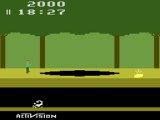Sam Trenholme's webpage
Support this website# More on Pitfall’s polynomial

## June 16 2013

In this blog, I continue looking at Pitfall's random number generator. This continues a series with three previous blog entries.

==How to make a LFSR more compact==

In a previous blog entry, I looked at how to implement a LFSR in 6502. The code took 18 bytes of ROM. The actual code used in the Pitfall cartridge uses 15 bytes:

uint8_t lfsr_3(uint8_t r) {
uint8_t a, c, t;
a = r;               // LDA r a: r
c = a >> 7; a <<= 1; // ASL a: r << 1
a ^= r;              // EOR r a: r << 1 ^ r
c = a >> 7; a <<= 1; // ASL a: r << 2 ^ r << 1
a ^= r;              // EOR r  a: r << 2 ^ r << 1 ^ r
c = a >> 7; a <<= 1; // ASL a: r << 3 ^ r << 2 ^ r << 1
c = a >> 7; a <<= 1; // ASL a: r << 4 ^ r << 3 ^ r << 2
a ^= r;              // EOR r  a: r << 4 ^ r << 3 ^ r << 2 ^ r

The next command is a little tricky. It takes the left bit of a and puts it in the “carry” bit.

When grabbing the 7th (left) bit of a value which is a series of left shifts, we can convert the left shifts in to the corresponding right shifts by taking 7 and subtracting it from the right shift value.

When this is done, grabbing the left bit makes a left shift of 0 become a right shift of 7, a left shift of 1 be a right shift of 6 and so on:

Before (<<)    After(>>)
0              7
1              6
2              5
3              4
4              3
5              2
6              1
7              0

This in mind, by taking the left bit of “r << 4 ^ r << 3 ^ r << 2 ^ r” (4,3,2,0), we get “r >> 3 ^ r >> 4 ^ r >> 5 ^ r >> 7” (3,4,5,7). Let’s continue the code in the actuall Pitfall cartridge:

c = a >> 7; a <<= 1; // ASL
t = r >> 7; r <<= 1; r |= c; c = t;     // ROL r
return r;
}

The reason David Crane wrote the code this way was to save three bytes; while the code is harder to follow, it’s now 15 instead of 18 bytes long.

==Why this particular tap sequence==

There are 16 different possible tap sequences for this particular random number generator. The reason Pitfall uses this particular one is because it has the most compact representation in 6502; other sequences use more code.

==More to come==

If David Crane knew then when we know today about pseudo-random number generators, he would have used a different algorithm. The code we have been looking at is actually called a “Fibonacci LFSR”. There is a variant way of representing a LFSR called a “Galois LFSR” which is even smaller; we will look at that as well as another small possible variant random number generator for Pitfall in a future blog entry.